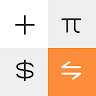# Mi Calculator

## Information

 Package com.miui.calculator Version 10.1.3 (100003) Updated 2019-04-24 Size 13 MB Installs 100,000,000 Category Tools

## Description

Mi Calculator will do the math for you. All new all-in-one calculator for you.
Regular, scientific, and mortgage calculators, as well as currency and unit converters and much more are all here in one place.

1. Basic calculator: Edit history and view previous results in the regular calculator.
2. Scientific calculator: Find trigonometric functions, logarithms, and more additional features in the scientific calculator.
3. Currency converter: Daily updated exchange rates in the universal currency converter.
4. Mortgage calculator: Know the exact amount of the next instalment with the loan/mortgage calculator.
5. Converters: Freely convert units of length, area, volume, speed, time, and mass in the unit converter
6. Age calculator: Calculate your age accurately and know more details about the birth date
7. GST calculator: Get the GST you are paying for your goods (For India)
8. BMI calculator: Stay fit by knowing your BMI
9. Discount calculator: Know the exact discount amount you are going to get
10. Percentage calculator: Precisely calculate the percentages with ease
11. Date calculator: Find difference between two dates
12. Split bill: Split the bills among friends and know the amount payable

## What's New

1. Fixed bugs
2. Optimised rendering of all calculators